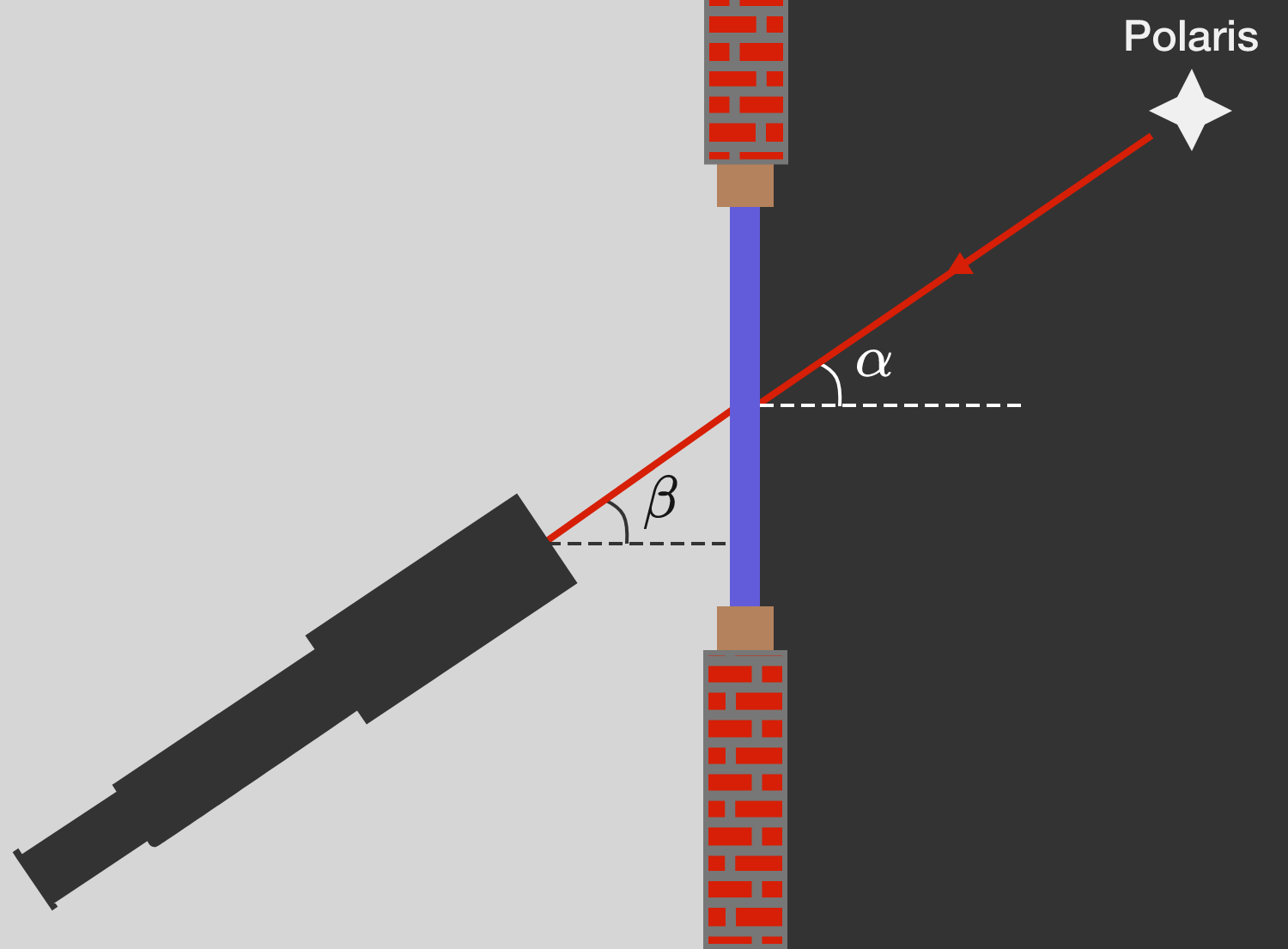# Angle measurement of Polaris

Galileo Gallei measured the angular positions of the stars with his telescope. To adjust his telescope, he initially aimed at the Pole Star, which is always at the same position in the sky at an angle of $\alpha$ with the horizontal.

On a cold winter's night of $\SI{-3}{\celsius},$ he conducted his measurements inside his study room at $\SI{20}{\celsius}.$ The starlight fell through his window and refracted twice on the glass. From his room, Polaris appeared at an angle of $\beta$ with the horizon.

What is the angle difference $\delta = \beta - \alpha$ between measurements inside and outside?Assumptions:

• Galileo's study room was in Venice, at a latitude of $\phi \approx \SI{45}{\degree}.$
• Air is an ideal gas with the specific gas constant $R_\text{air}^\ast \approx 300 \text{ J}/(\text{kg} \cdot \text{K}).$
• Air is a dielectric with an electric susceptibility of $\chi_\text{air} = \varepsilon_\text{air} - 1 = c \cdot \rho_\text{air}\, \big(\text{where } c \approx 10^{-3} \text{ m}^3/\text{kg}\big).$
×

Problem Loading...

Note Loading...

Set Loading...